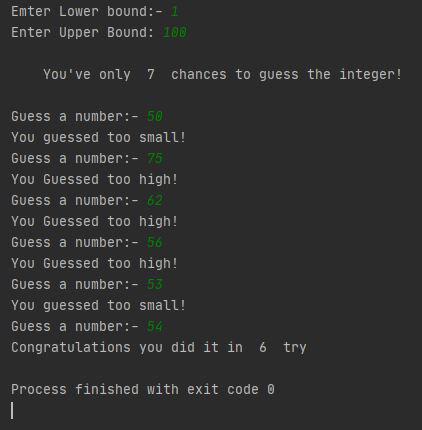# Python 3 和 C 中的猜数字游戏

• 构建一个猜数字游戏，用户在其中选择一个范围。
• 假设用户选择了一个范围，即从AB，其中AB属于 Integer。
• 系统将选择一些随机整数，用户必须在最小猜测次数内猜测该整数

Minimum number of guessing = log2(Upper bound – lower bound + 1)

### 算法：以下是步骤：

• 用户输入范围的下限上限。
• 编译器生成该范围之间的随机整数并将其存储在变量中以供将来参考。
• 对于重复猜测，将初始化一个 while 循环。
• 如果用户猜测的数字大于随机选择的数字，用户会得到一个输出“再试一次！” 你猜得太高了
• 否则，如果用户猜测的数字小于随机选择的数字，则用户会得到输出“再试一次！” 你猜得太小了”
• 如果用户猜中了最少的猜测次数，则用户会得到“恭喜！“ 输出。
• 否则，如果用户没有在最小猜测次数中猜出整数，他/她将得到“下次好运！” “ 输出。

• Python3
• C
``````import random
import math
# Taking Inputs
lower = int(input("Enter Lower bound:- "))

# Taking Inputs
upper = int(input("Enter Upper bound:- "))

# generating random number between
# the lower and upper
x = random.randint(lower, upper)
print("\n\tYou've only ",
round(math.log(upper - lower + 1, 2)),
" chances to guess the integer!\n")

# Initializing the number of guesses.
count = 0

# for calculation of minimum number of
# guesses depends upon range
while count < math.log(upper - lower + 1, 2):
count += 1

# taking guessing number as input
guess = int(input("Guess a number:- "))

# Condition testing
if x == guess:
print("Congratulations you did it in ",
count, " try")
# Once guessed, loop will break
break
elif x > guess:
print("You guessed too small!")
elif x < guess:
print("You Guessed too high!")

# If Guessing is more than required guesses,
# shows this output.
if count >= math.log(upper - lower + 1, 2):
print("\nThe number is %d" % x)
print("\tBetter Luck Next time!")

# Better to use This source Code on pycharm!``````